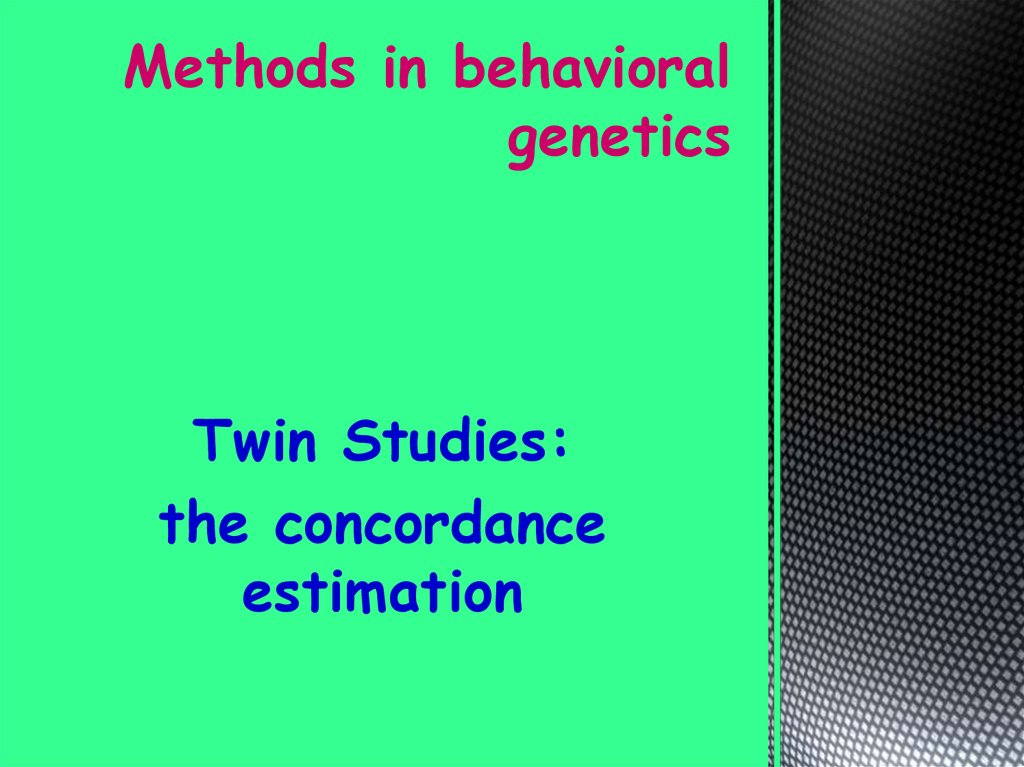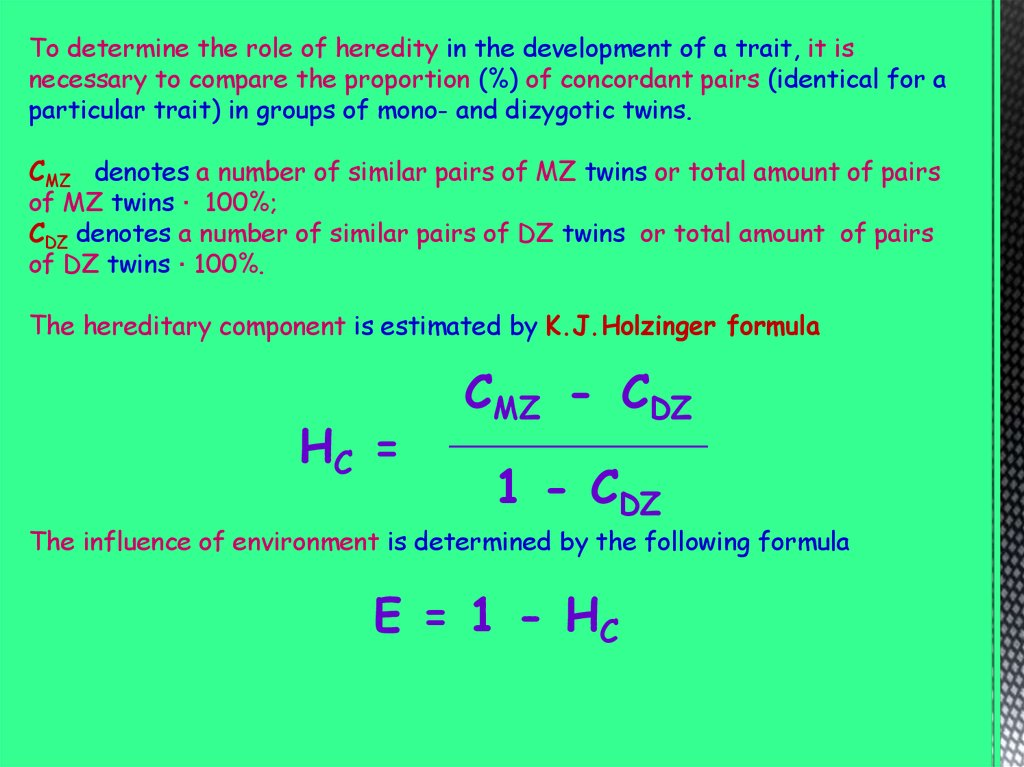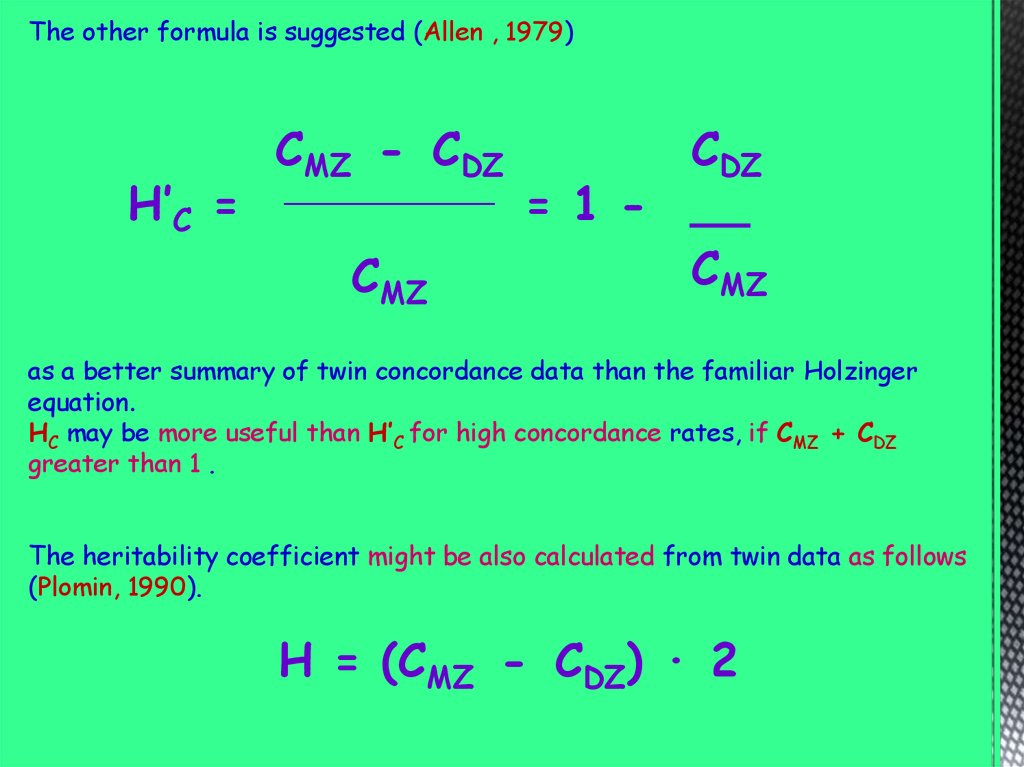# Methods in behavioral genetics. Twin Studies: the concordance estimation

Twin Studies:
the concordance
estimation

## 2.

To determine the role of heredity in the development of a trait, it is
necessary to compare the proportion (%) of concordant pairs (identical for a
particular trait) in groups of mono- and dizygotic twins.
CMZ denotes a number of similar pairs of MZ twins or total amount of pairs
of MZ twins · 100%;
CDZ denotes a number of similar pairs of DZ twins or total amount of pairs
of DZ twins · 100%.
The hereditary component is estimated by K.J.Holzinger formula
HC =
CMZ - CDZ
________________
1 - CDZ
The influence of environment is determined by the following formula
E = 1 - HC

## 3.

The other formula is suggested (Allen , 1979)
H’C =
CMZ - CDZ
_____________
CMZ
CDZ
= 1 - __
CMZ
as a better summary of twin concordance data than the familiar Holzinger
equation.
HC may be more useful than H’C for high concordance rates, if CMZ + CDZ
greater than 1 .
The heritability coefficient might be also calculated from twin data as follows
(Plomin, 1990).
H = (CMZ - CDZ) · 2

## 4.

Problem
Concordance of MZ twins in tuberculosis is 66.7%, and DZ ones is 23%.
What are both shares of heredity and the environment in the development
of the disease?
The classical Holzinger formula allows to calculate the share of heredity (H)
and the environment (E) in the development of the trait under study.
CMZ = 0.667
CDZ = 0.23
HC =
0.667 - 0.23
_____________ =
1 - 0.23
0.437
_____
= 0.567
0.77
E = 1 – HC = 1 – 0.657 = 0.433
Calculations have shown that hereditary factors are essential in the
development of this pathology.
The share of heredity in the development of tuberculosis is 0.567;
the share of the environment in the development of this disease is 0.433.

## 5.

Problem
The concordance of MZ twins in schizophrenia is 79%, and DZ ones is
4.4%.
What are the shares of heredity and the environment in the
development of schizophrenia?
Using classical Holzinger formula me can calculate the share of heredity (H)
and the environment (E) in the development of the disorder.
CMZ = 0.79
CDZ = 0.044
HC =
0.79 - 0.044
_____________ =
1 - 0.044
0.746
_____
= 0.78
0.956
E = 1 – HC = 1 – 0.78 = 0.22
Calculations have shown that hereditary factors are essential in the
development of this patholog
The share of heredity in the development of tuberculosis is 0.78;
the share of the environment in the development of this disease is 0.22.

## 6.

Intelligence Genes: Are there Genius Genes?

## 8.

The method of adoptive children is considered the purest one of behavioral
genetics with maximum resolution
It is based on a simple principle:
parents are included in the study as soon as possible.
The children possess about 50% of the total number of genes with their
biological parents, as close relatives, but do not of the general environment.
With the adoptive ones they have a common environment, but do not
common genes.
If hereditary determination is stronger, greater resemblance to biological
parents is expected.
If the result is the opposite, then this is due to the predominance of the
influence of the environment, and the children will be similar to a foster
parents.

## 9.

There are two variants of this method: complete and partial
The complete one analyzes the combined data from adoptive siblings and two
groups of separated relatives, such as parents and their biological children,
given to adoption, and separated siblings, who all undergo an intergroup
comparison.
Study of these three groups allows to identify reliably the factors that form
the family resemblance.
Partial method is a research that is carried out with adoptive and separated
siblings.
To provide the representability of the study it should include
- the wide range of conditions in adoptive families and
- some substantial features which are similar , e.g. intelligence or nurture
style of parents.

## 10.

One of disadvantages of the adoption studies is the problem, whether the
group of women that gives children away may be considered adequate to
population.
The other problem is the characteristics of a new family. If they resemble
the native one the correlation will be distorted.
The third point is a maternal affect on a child in uterus. The influence is
environmental to the fetus and raises the similarity of the mother and the
child.

## 11.

Twin studies and adoption studies (10 min)

## 12. Methods in behavioral genetics

Population Studies

## 13.

In biology population is ‘the number of organisms of the same species that live
in a particular geographic area at the same time, with the capability of random
mating’.
This method of behaviour genetics is aimed at studying the inheritance of
mental disorders in the families and it comparing to the frequency of the
disease among the population living in similar conditions.
The human population has a number of demographic parameters:
size, birth and mortality rate, age structure, sex structure, occupation of its
members, economic status, geographical and climatic living conditions, etc.
Small populations of between 1,500 and 4,000 people are called demes. They
are characterized by a small proportion of people of other origin (1-2%), a
high frequency of intragroup marriages (80-90%), a slight increase in
population (about 20% in 25 years).
Groups of no more than 1,500 people are called isolates. The share of
foreigners in them does not exceed 1%, the frequency of intra-group
marriages is more than 90%, the natural increase over 25 years is less than
25%. If the isolate persists for 4 generations (about 100 years), then all its
members become at least second cousins.

## 14.

There are some peculiarities in populations of human.
Modern human populations are characterized by two traits:
1) an increase in numbers;
2) a decrease in natural selection pressure.
In economically developed countries mortality has significantly decreased,
which has shifted the focus of the selection to reproductive selectivity.
Reducing the number of children in families has led to a narrowing of the
field of selection.
In addition, modern human populations tend to
a) the destruction of marital isolates,
b) homogenization of the environment leads to the disappearance of causes
of racial differences,
c) improvement of the environment that allows for full genotypic
manifestation of the traits such as height, maturation rate, etc.,
d) replacement of the main causes of death by others, e.g. cardiovascular and
oncological diseases instead of infectious and alimentary ones.

## 15.

Mechanisms to maintain the genetic structure of populations
The genetic structure of the population is determined by the system of
marriages and factors that change the frequency of genes.
Therefore, those mechanisms that maintain gene equilibrium or alter it are
extremely important.
Stabilization of the frequency ratio of alleles in a number of generations
obeys the Hardy-Weinberg equilibrium proposed in 1908.
The law states that allele and genotype frequencies in a population will remain
constant from generation to generation in the absence of other evolutionary
influences.
There are seven assumptions underlying Hardy–Weinberg equilibrium:
- organisms are diploid;
- only sexual reproduction occurs;
- generations have no overlapping;
- mating is random;
- population size is infinitely large;
- allele frequencies are equal in the sexes;
- there is no migration, gene flow, admixture, mutation or selection.
Deviations in proportion indicate the manifestation of certain population
processes.

## 16.

The simplest case is a single locus with two alleles denoted A (dominant) and
a (recessive).
The frequency of A is called p and the same of a - q.
The sum frequency of both alleles is equal to 1
p + q = 1
To calculate the expected frequency of genotypes should use the Punnet
square.
Phenotype
A
a
Genotype
p
A
p
(AA) p2
a
q
(Aa) pq
q
(Aa) pq
(aa) q2

## 17.

The resulting equation is
p2 + 2pq + q2 = 1
or
(p = q)2 = 1
To estimate the expected phenotypes frequencies it is necessary to change
parts of the equilibrium
p2 = P q2 = Q 2pq =H
P +H + Q = 1

## 18.

The Hardy-Weinberg Principle: Watch your Ps and Qs (12 min)
How to solve Hardy Weinberg problems (9 min)
Hardy-Weinberg practice problems

## 19.

Problem
What is the frequency of heterozygotes Aa in a randomly mating population
in which the frequency of all dominant phenotypes is 0.19?
Here (dominant phenotypes)
applying the law
we know that
p2 + 2pq = 0.19
q2 = 1 - 0.19 = 0.81
q = 0.9
p + q = 1,
then
here
p = 1 – q
p = 1-q = 0.1
Frequency of heterozygotes
Aa (2pq) = 2(0.1 ·0.9) = 0.18

## 20.

Problem
αβγ is autosomal recessive mental disorder of human. The frequency of
affected newborn infants is about 1 in 14,000.
Assuming random mating, what is the frequency of unaffected carriers?
Here
q2 = 1/14,000
q2 = 0.000071428
q = 0.0085
we know that
then
here
p + q = 1,
p = 1- q = 1 – 0.0085
Frequency of carriers
p = 0.9915
Aa (2pq) = 2(0.9915 ·0.0085) = 0.017

## 21.

Problem
In a small village in Africa, 15% of the population is
born with sickle-cell anemia (aa). (q2=0.15)
1.What is the frequency for the “A” allele?
p = 1 - 0.3873 = 0.6127
2.What is the frequency for the “a” allele?
q = 0.3873
3.What are the three genotype frequencies?
AA = p2 = 0(.6127)2= 0.3754
Aa = 2pq = 2(0.6127)(0.3873) =0.4746
aa =0.15
4. Assuming Hardy-Weinberg equilibrium, how many
individuals in a neighboring village of 3,500 would be
expected to be heterozygous?
3,500(0.4746) = 1661.1 = 1661 individuals

## 22.

Hardy–Weinberg proportions for two alleles: the horizontal axis shows the two
allele frequencies p and q and the vertical axis shows the expected genotype
frequencies. Each line shows one of the three possible genotypes.

## 23.

Equilibrium of genes by one autosomal allele is achieved through one generation
and persists further if factors that disturb the balance do not intervene.
The proportion of heterozygotes in the population can not be over than 50% (if
p = q = 0.5, then P + Q = H = 0.5).
Also, the number of heterozygotes can not be more than the frequency of one
type of homozygotes.
The Hardy-Weinberg law can also be used for multiallel genes
(p + q + r + ... + s)2 = 1
For the calculation of alleles for blood groups ABO, the scheme will be:
p = √(i +IA), q = √(i +IB), r = √i,
where the frequencies are: p is IA, q is IB , and r is i.

## 24.

Genes, environment and responsibility for behavior (42 min)